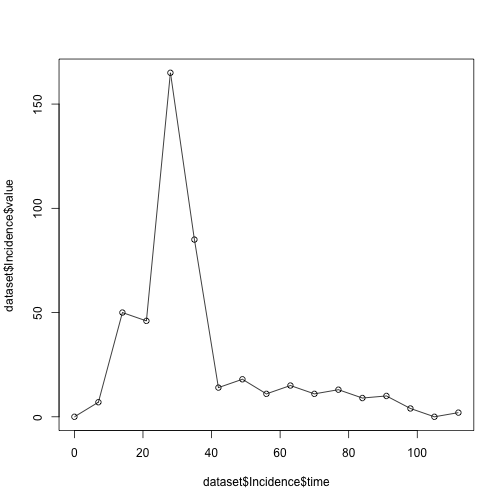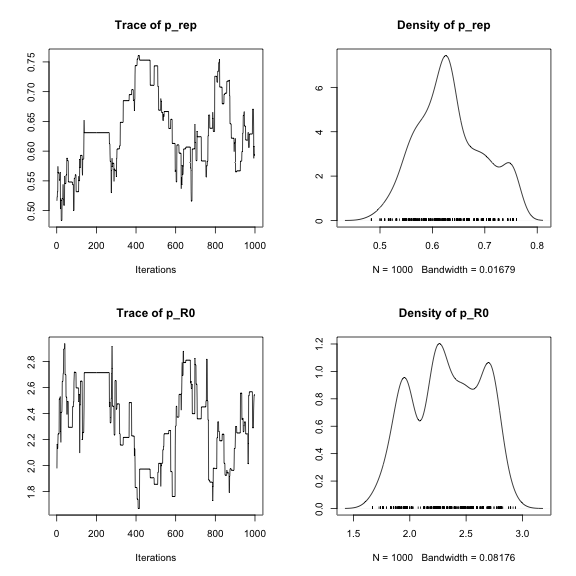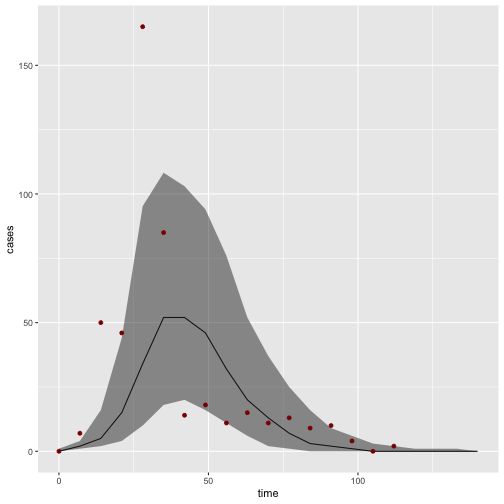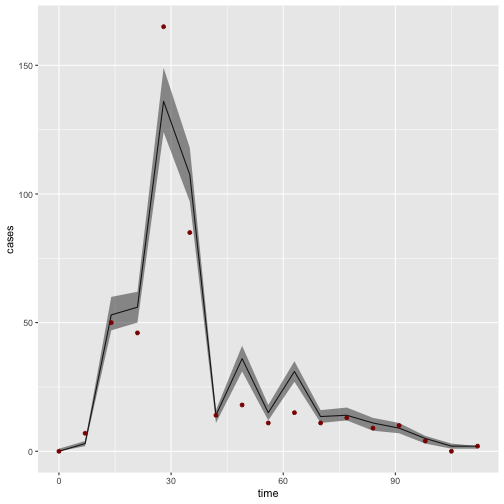# Introduction to RBi

Sebastian Funk on July 10, 2019

RBi is an R interface to LibBi, a library for Bayesian inference with state-space models using high-performance computer hardware.

The package has been tested on macOS/OSX and Linux. It requires a working installation of LibBi. One macOS, this is easiest done using the the brew command: Install Homebrew, then issue the following command (using a command shell, i.e. Terminal or similar):

brew install libbi


On linux, follow the instructions provided with LibBii.

If you have any trouble installing LibBi you can get help on the LibBi Users mailing list.

The path to the libbi script can be passed as an argument to RBi, otherwise the package tries to find it automatically using the which linux/unix command.

If you just want to process the output from LibBi, then you do not need to have LibBi installed.

# Installation

The RBi package requires R (>= 3.2.0) as well as the packages:

• reshape2
• ncdf4
• data.table

The easiest way to install the latest stable version of RBi is via CRAN. The package name is rbi (all lowercase):

install.packages('rbi')


Alternatively, the current development version can be installed using the devtools package

# install.packages("devtools")
library('devtools')
install_github("sbfnk/rbi")


Use

library('rbi')


to load the package.

# Getting started

The main computational engine and model grammar behind RBi is provided by LibBi. The LibBi manual is a good place to start for finding out everything there is to know about LibBi models and inference methods.

The RBi package mainly provides two classes: bi_model and libbi. The bi_model class is used to load, view and manipulate LibBi model files. The libbi class is used to run LibBi and perform inference.

The package also provides two methods for interacting with the NetCDF files used by LibBi, bi_read and bi_write. Lastly, it provides a get_traces function to analyse Markov-chain Monte Carlo (MCMC) traces using the coda package.

# The bi_model class

As an example, we consider a simplified version of the SIR model discussed in Del Moral et al. (2014). This is included with the RBi package and can be loaded with

model_file <- system.file(package="rbi", "SIR.bi")
SIRmodel <- bi_model(model_file) # load model


Other ways of implementing a (deterministic or stochastic) SIR model can be found in the collection of SIR models for LibBi, where you also find how to load them into a bi_model object, e.g. SIRmodel. Feel free to run the commands below with different versions of the model.

The SIRmodel object now contains the model, which can be displayed with

SIRmodel

## bi_model:
## =========
##  1: model SIR {
##  2:   const h = 7
##  3:   const N = 1000
##  4:   const d_infection = 14
##  5:   noise n_transmission
##  6:   noise n_recovery
##  7:   state S
##  8:   state I
##  9:   state R
## 10:   state Z
## 11:   obs Incidence
## 12:   param p_rep
## 13:   param p_R0
## 14:   sub parameter {
## 15:     p_rep ~ uniform(0,1)
## 16:     p_R0 ~ uniform(1,3)
## 17:   }
## 18:   sub initial {
## 19:     S <- N - 1
## 20:     I <- 1
## 21:     R <- 0
## 22:     Z <- 1
## 23:   }
## 24:   sub transition {
## 25:     n_transmission ~ wiener()
## 26:     n_recovery ~ wiener()
## 27:     Z <- (t_now % 7 == 0 ? 0 : Z)
## 28:     inline i_beta = p_R0 / d_infection * exp(n_transmission)
## 29:     inline i_gamma = 1 / d_infection * exp(n_recovery)
## 30:     ode (alg='RK4(3)', h=1e-1, atoler=1e-2, rtoler=1e-5) {
## 31:       dS/dt = - i_beta * S * I / N
## 32:       dI/dt = i_beta * S * I / N - i_gamma * I
## 33:       dR/dt = i_gamma * I
## 34:       dZ/dt = i_beta * S * I / N
## 35:     }
## 36:   }
## 37:   sub observation {
## 38:     Incidence ~ poisson(p_rep * Z)
## 39:   }
## 40:   sub proposal_initial {
## 41:     S <- N - 1
## 42:     I <- 1
## 43:     R <- 0
## 44:     Z <- 1
## 45:   }
## 46:   sub proposal_parameter {
## 47:     p_rep ~ truncated_gaussian(mean = p_rep, std = 0.03, lower = 0, upper = 1)
## 48:     p_R0 ~ truncated_gaussian(mean = p_R0, std = 0.2, lower = 1, upper = 3)
## 49:   }
## 50: }


A part of the model can be shown with, for example,

SIRmodel[35:38]

##  "}"                              "}"
##  "sub observation {"              "Incidence ~ poisson(p_rep * Z)"


or, for example,

get_block(SIRmodel, "parameter")

##  "p_rep ~ uniform(0,1)" "p_R0 ~ uniform(1,3)"


To get a list of certain variables, you can use the var_names function. For example, to get a list of states, you can use

var_names(SIRmodel, type="state")

##  "S" "I" "R" "Z"


There are also various methods for manipulating a model, such as remove_lines, insert_line, replace_all.

The fix method fixes a variable to one value. This can be useful, for example, to run the deterministic equivalent of a stochastic model for testing purposes:

det_SIR_model <- fix(SIRmodel, n_transmission=0, n_recovery=0)


To get documentation for any of these methods, use the links in the documentation for bi_model.

# Generating a dataset

First, let's create a data set from the SIR model.

set.seed(1001912)
SIRdata <- bi_generate_dataset(SIRmodel, end_time=16*7, noutputs=16)


This simulates the model a single time from time 0 until time 16*7 (say, 16 weeks with a daily time step), producing 16 outputs (one a week). Note that we have specified a random seed to make this document reproducible. If you omit the set.seed command or set it to a different number, the results will be different even when run with the same set of commands. Also note that LibBi compiles the model code only the first time it is run. If you run the command above a second time, it should run much faster.

The bi_generate_dataset function returns a libbi object:

SIRdata

## Wrapper around LibBi
## ======================
## Model:  SIR
## Run time:  0.001299  seconds
## Number of samples:  1
## State trajectories recorded:  S I R Z
## Noise trajectories recorded:  n_transmission n_recovery
## Observation trajectories recorded:  Incidence
## Parameters recorded:  p_rep p_R0


The generated dataset can be viewed and/or stored in a variable using bi_read:

dataset <- bi_read(SIRdata)


The bi_read function takes the name of a NetCDF file or a libbi object (in which case it locates the output file) and stores the contents in a list of data frames or vectors, depending on the dimensionality of the contents. Note that, if no working_folder is specified, the model and output files will be stored in a temporary folder.

names(dataset)

##   "n_transmission" "n_recovery"     "S"              "I"
##   "R"              "Z"              "Incidence"      "p_rep"
##   "p_R0"           "clock"

dataset$p_R0  ## value ## 1 2.159818  dataset$Incidence

##    time value
## 1     0     0
## 2     7     7
## 3    14    50
## 4    21    46
## 5    28   165
## 6    35    85
## 7    42    14
## 8    49    18
## 9    56    11
## 10   63    15
## 11   70    11
## 12   77    13
## 13   84     9
## 14   91    10
## 15   98     4
## 16  105     0
## 17  112     2


We can visualise the generated incidence data with

plot(dataset$Incidence$time, dataset$Incidence$value)
lines(dataset$Incidence$time, dataset$Incidence$value)# The libbi class

The libbi class manages the interaction with LibBi such as sampling from the prior or posterior distribution. For example, the SIRdata object above is of type libbi:

class(SIRdata)

##  "libbi"


Th bi_generate_dataset is one particular way of generating a libbi object, used only to generate test data from a model. The standard way of creating a libbi object for Bayesian inference is using the libbi command

bi <- libbi(SIRmodel)


This initialises a libbi object with the model created earlier and assigns it to the variable bi.

class(bi)

##  "libbi"


Let's sample from the prior of the SIR model:

bi_prior <- sample(bi, target="prior", nsamples=1000, end_time=16*7, noutputs=16)


This step calls LibBi to sample from the prior distribution of the previously specified model, generating 1,000 samples and each time running the model for 16 * 7 = 112 time steps and writing 16 outputs (i.e., every 7 time steps). LibBi parses the model, creates C++ code, compiles it and run the model. If the model is run again, it should do so much quicker because it will use the already compiled C++ code to run the model:

bi_prior <- sample(bi_prior)


The sample command returns an updated libbi object which, in this case, we just assign again to the bi object. Any call of sample preserves options passed to the previous call of sample and libbi, unless they are overwritten by arguments passed to sample (e.g., passing a new nsamples argument). Let's have a closer look at the bi object:

bi_prior

## Wrapper around LibBi
## ======================
## Model:  SIR
## Run time:  0.253129  seconds
## Number of samples:  1000
## State trajectories recorded:  S I R Z
## Noise trajectories recorded:  n_transmission n_recovery
## Parameters recorded:  p_rep p_R0


To see even more detail, try

str(bi_prior)

## List of 22
##  $options :List of 4 ## ..$ seed    : num 3.33e+08
##   ..$nsamples: num 1000 ## ..$ end-time: num 112
##   ..$noutputs: num 16 ##$ path_to_libbi   : chr "/usr/local/bin/libbi"
##  $model : 'bi_model' chr [1:50] "model SIR {" "const h = 7" "const N = 1000" "const d_infection = 14" ... ##$ model_file_name : chr "/private/var/folders/qx/7hd7ngzn6d72tnb478f3mg2h0000gn/T/Rtmpelo1ne/SIR1181b60976d91/SIR.bi"
##  $working_folder : chr "/var/folders/qx/7hd7ngzn6d72tnb478f3mg2h0000gn/T//Rtmpelo1ne/SIR1181b60976d91" ##$ dims            : list()
##  $time_dim : chr(0) ##$ coord_dims      : list()
##  $thin : num 1 ##$ output_every    : num NA
##  $debug : logi FALSE ##$ command         : chr "/usr/local/bin/libbi sample --seed 332755949 --target prior --nsamples 1000 --end-time 112 --noutputs 16 --outp"| __truncated__
##  $output_file_name: chr "/private/var/folders/qx/7hd7ngzn6d72tnb478f3mg2h0000gn/T/Rtmpelo1ne/SIR1181b60976d91/SIR_output1181b6c275f71.nc" ##$ log_file_name   : chr "/private/var/folders/qx/7hd7ngzn6d72tnb478f3mg2h0000gn/T/Rtmpelo1ne/SIR1181b60976d91/output1181b7c6f4149.txt"
##  $user_log_file : logi FALSE ##$ timestamp       :List of 1
##   ..$output: POSIXct[1:1], format: "2019-07-10 11:18:47" ##$ run_flag        : logi TRUE
##  $error_flag : logi FALSE ##$ use_cache       : logi TRUE
##  $supplement : NULL ##$ .gc_env         :<environment: 0x7fbead580a38>
##  $.cache :<environment: 0x7fbeaf326a80> ## - attr(*, "class")= chr "libbi"  We can see the object contains 14 fields, including the model, the path to the libbi script, and the command used to run libbi (bi$command); the options field contains all the options that LibBi was called with. This includes the ones we passed to sample

bi_prior$options  ##$seed
##  332755949
##
## $nsamples ##  1000 ## ##$end-time
##  112
##
## $noutputs ##  16  The other fields contain various bits of information about the object, including the model used, the command used to run LibBi (bi$command) and the output file name:

bi_prior$output_file_name  ##  "/private/var/folders/qx/7hd7ngzn6d72tnb478f3mg2h0000gn/T/Rtmpelo1ne/SIR1181b60976d91/SIR_output1181b6c275f71.nc"  We can get the results of the sampling run using bi_read prior <- bi_read(bi_prior$output_file_name)


or with the shorthand

prior <- bi_read(bi_prior)


which looks at the output_file_name field to read in the data. Let's look at the returned object

str(prior)

## List of 9
##  $n_transmission:'data.frame': 17000 obs. of 3 variables: ## ..$ np   : num [1:17000] 0 0 0 0 0 0 0 0 0 0 ...
##   ..$time : num [1:17000] 0 7 14 21 28 35 42 49 56 63 ... ## ..$ value: num [1:17000] 0 -0.2789 -0.0801 -0.08 -0.5102 ...
##  $n_recovery :'data.frame': 17000 obs. of 3 variables: ## ..$ np   : num [1:17000] 0 0 0 0 0 0 0 0 0 0 ...
##   ..$time : num [1:17000] 0 7 14 21 28 35 42 49 56 63 ... ## ..$ value: num [1:17000] 0 -0.562 0.857 1.3 1.51 ...
##  $S :'data.frame': 17000 obs. of 3 variables: ## ..$ np   : num [1:17000] 0 0 0 0 0 0 0 0 0 0 ...
##   ..$time : num [1:17000] 0 7 14 21 28 35 42 49 56 63 ... ## ..$ value: num [1:17000] 999 993 970 801 664 ...
##  $I :'data.frame': 17000 obs. of 3 variables: ## ..$ np   : num [1:17000] 0 0 0 0 0 0 0 0 0 0 ...
##   ..$time : num [1:17000] 0 7 14 21 28 35 42 49 56 63 ... ## ..$ value: num [1:17000] 1 5.1 20.8 129.8 115.6 ...
##  $R :'data.frame': 17000 obs. of 3 variables: ## ..$ np   : num [1:17000] 0 0 0 0 0 0 0 0 0 0 ...
##   ..$time : num [1:17000] 0 7 14 21 28 35 42 49 56 63 ... ## ..$ value: num [1:17000] 0 2.16 8.9 69.02 219.96 ...
##  $Z :'data.frame': 17000 obs. of 3 variables: ## ..$ np   : num [1:17000] 0 0 0 0 0 0 0 0 0 0 ...
##   ..$time : num [1:17000] 0 7 14 21 28 35 42 49 56 63 ... ## ..$ value: num [1:17000] 1 6.27 22.39 169.19 136.75 ...
##  $p_rep :'data.frame': 1000 obs. of 2 variables: ## ..$ np   : num [1:1000] 0 1 2 3 4 5 6 7 8 9 ...
##   ..$value: num [1:1000] 0.966 0.546 0.539 0.109 0.767 ... ##$ p_R0          :'data.frame':  1000 obs. of  2 variables:
##   ..$np : num [1:1000] 0 1 2 3 4 5 6 7 8 9 ... ## ..$ value: num [1:1000] 1.7 1.82 2.19 2.97 1.38 ...
##  $clock : num 253129  This is a list of 9 objects, 8 representing each of the (noise/state) variables and parameters in the file, and one number clock, representing the time spent running the model in microseconds. We can see that the time-varying variables are represented as data frames with three columns: np (enumerating individual simulations), time and value. Parameters don't vary in time and just have np and value columns. # Fitting a model to data using PMCMC Let's perform inference using Particle Markov-chain Metropolis Hastings (PMMH). The following command will generate 16 * 10,000 = 160,000 simulations and therefore may take a little while to run (if you want to see the samples progress, use verbose=TRUE in the sample call). bi <- sample(bi_prior, target="posterior", nparticles=32, obs=SIRdata)  This samples from the posterior distribution. Remember that options are preserved from previous runs (because we passed the bi) as first argument, so we don't need to specify nsamples, end_time and noutputs again, unless we want to change them. The nparticles option specifies the number of particles. You can also pass a list of data frames (each element of the list corresponding to one observed variable as the obs argument, for example df <- data.frame(time = c(0,7,14,21,28,35,42,49,56,63,70,77,84,91,98,105,112), value = c(1,6,2,26,99,57,78,57,15,9,4,1,1,1,0,2,0)) bi_df <- sample(bi_prior, target="posterior", nparticles=32, obs=list(Incidence=df))  Input, init and observation files (see the LibBi manual for details) can be specified using the init, input, obs options, respectively. They can each be specified either as the name of a NetCDF file containing the data, or a libbi object (in which case the output file will be taken) or directly via an appropriate R object containing the data (e.g., a character vector of length one, or a list of data frames or numeric vectors). In the case of the command above, init is specified as a list, and obs as a libbi object. The Incidence variable of the SIRdata object will be taken as observations. The time dimension (or column, if a data frame) in the passed init, input and/or obs files can be specified using the time_dim option. If this is not given, it will be assumed to be time, if such a dimension exists or, if not, any numeric column not called value (or the contents of the value_column option). If this does not produce a unique column name, an error will be thrown. All other dimensions/columns in the passed options will be interpreted as additional dimensions in the data, and stored in the dims field of the libbi object. Any other options (apart from log_file_name, see the Debugging section) will be passed on to the command libbi – for a complete list, see the LibBi manual. Hyphens can be replaced by underscores so as not to confuse R (see end_time). Any arguments starting with enable/disable can be specified as boolean (e.g., assert=TRUE). Any dry- options can be specified with a "dry" argument, e.g., parse="dry". # Analysing an MCMC run Let's get the results of the preceding sample command: bi_contents(bi)  ##  "time" "n_transmission" "n_recovery" "S" ##  "I" "R" "Z" "p_rep" ##  "p_R0" "clock" "loglikelihood" "logprior"  posterior <- bi_read(bi) str(posterior)  ## List of 11 ##$ n_transmission:'data.frame':  17000 obs. of  3 variables:
##   ..$np : num [1:17000] 0 0 0 0 0 0 0 0 0 0 ... ## ..$ time : num [1:17000] 0 7 14 21 28 35 42 49 56 63 ...
##   ..$value: num [1:17000] 0 -0.90631 -1.42948 -0.00768 2.00952 ... ##$ n_recovery    :'data.frame':  17000 obs. of  3 variables:
##   ..$np : num [1:17000] 0 0 0 0 0 0 0 0 0 0 ... ## ..$ time : num [1:17000] 0 7 14 21 28 35 42 49 56 63 ...
##   ..$value: num [1:17000] 0 0.1069 1.6124 -0.011 -0.0693 ... ##$ S             :'data.frame':  17000 obs. of  3 variables:
##   ..$np : num [1:17000] 0 0 0 0 0 0 0 0 0 0 ... ## ..$ time : num [1:17000] 0 7 14 21 28 35 42 49 56 63 ...
##   ..$value: num [1:17000] 999 994 910 821 605 ... ##$ I             :'data.frame':  17000 obs. of  3 variables:
##   ..$np : num [1:17000] 0 0 0 0 0 0 0 0 0 0 ... ## ..$ time : num [1:17000] 0 7 14 21 28 35 42 49 56 63 ...
##   ..$value: num [1:17000] 1 3.96 52.18 92.21 229.24 ... ##$ R             :'data.frame':  17000 obs. of  3 variables:
##   ..$np : num [1:17000] 0 0 0 0 0 0 0 0 0 0 ... ## ..$ time : num [1:17000] 0 7 14 21 28 35 42 49 56 63 ...
##   ..$value: num [1:17000] 0 1.87 38.27 87.13 166.03 ... ##$ Z             :'data.frame':  17000 obs. of  3 variables:
##   ..$np : num [1:17000] 0 0 0 0 0 0 0 0 0 0 ... ## ..$ time : num [1:17000] 0 7 14 21 28 35 42 49 56 63 ...
##   ..$value: num [1:17000] 1 4.83 84.61 88.9 215.92 ... ##$ p_rep         :'data.frame':  1000 obs. of  2 variables:
##   ..$np : num [1:1000] 0 1 2 3 4 5 6 7 8 9 ... ## ..$ value: num [1:1000] 0.516 0.523 0.523 0.532 0.532 ...
##  $p_R0 :'data.frame': 1000 obs. of 2 variables: ## ..$ np   : num [1:1000] 0 1 2 3 4 5 6 7 8 9 ...
##   ..$value: num [1:1000] 1.98 2.16 2.16 2.13 2.13 ... ##$ clock         : num 9542550
##  $loglikelihood :'data.frame': 1000 obs. of 2 variables: ## ..$ np   : num [1:1000] 0 1 2 3 4 5 6 7 8 9 ...
##   ..$value: num [1:1000] -73.2 -63 -63 -61.4 -61.4 ... ##$ logprior      :'data.frame':  1000 obs. of  2 variables:
##   ..$np : num [1:1000] 0 1 2 3 4 5 6 7 8 9 ... ## ..$ value: num [1:1000] -0.693 -0.693 -0.693 -0.693 -0.693 ...


We can see that this has two more objects than previously when we specified target="prior": loglikelihood (the estimated log-likelihood of the parameters at each MCMC step) and logprior (the estimated log-prior density of the parameters at each MCMC step).

To get a summary of the parameters sampled, use

summary(bi)

##     var      Min.   1st Qu.    Median      Mean   3rd Qu.     Max.
## 1 p_rep 0.4834458 0.5867194 0.6309827 0.6340941 0.6795422 0.760625
## 2  p_R0 1.6692232 2.0160663 2.3031503 2.3281296 2.5961652 2.937201


A summary of sampled trajectories can be obtained using

summary(bi, type="state")


Any particular posterior sample can be viewed with extract_sample (with indices running from 0 to (nsamples-1)):

extract_sample(bi, 314)


To analyse MCMC outputs, we can use the coda package and the get_traces function of RBi. Note that, to get exactly the same traces, you would have to set the seed as above.

library('coda')
traces <- mcmc(get_traces(bi))


We can, for example, visualise parameter traces and densities with

plot(traces)Compare this to the marginal posterior distributions to the “correct” parameters used to generate the data set:

bi_read(SIRdata, type="param")

## $p_rep ## value ## 1 0.5093273 ## ##$p_R0
##      value
## 1 2.159818


For more details on using coda to further analyse the chains, see the website of the coda package. For more plotting functionality, the ggmcmc package is also worth considering.

# Predictions

We can use the predict function to re-simulate the fitted model using the estimated parameters, that is to generate samples from $$p(x_t|\theta)$$ where the $$\theta$$ are distributed according to the marginal posterior distribution $$p(\theta|y^*_t)$$ (here: $$\theta$$ are fixed parameters, $$x_t$$ are state trajectories and $$y^*_t$$ observed data points, as in the LibBi manual). This can be useful, for example, for comparing typical model trajectories to the data, or for running the model beyond the last data point.

pred_bi <- predict(bi, start_time=0, end_time=20*7, output_every=7, with=c("transform-obs-to-state")


where with=c("transform-obs-to-state") tells LibBi to treat observations as a state variable, that is to randomly generate observations, i.e. samples from $$p(y_t|\theta)$$ where, again, $$theta$$ are distributed according to the posterior distribution $$p(\theta|y^*_t)$$ (see the with-transform-obs-to-state option in the LibBi manual).

# Sample observations

To sample observations from sampled posterior state trajectories, that is samples from $$p(y_t|x_t)$$ where the $$x_t$$ are distributed according to the posterior distribution $$p(x_t | y_t)$$, you can use.

obs_bi <- sample_obs(bi)


Compare this to the data:

summary(obs_bi, type="obs")
dataset$Incidence  # Filtering The other so-called clients of LibBi (besides sample) are supported through commands of the same name:filter, optimise and rewrite. For example, to run a particle filter on the last posterior sample generated above, you can use: bi_filtered <- filter(bi)  # Plotting Output form LibBi runs can be visualised using standard R plotting routines or plotting packages such as ggplot2. The summary function can help with this. For example, to plot observations randomly generated from the posterior distribution of the parameters and compare them to the data we can use ps <- summary(pred_bi, type="obs") library('ggplot2') ggplot(ps, aes(x=time))+ geom_line(aes(y=Median)) + geom_ribbon(aes(ymin=1st Qu., ymax=3rd Qu.), alpha=0.5) + geom_point(aes(y=value), dataset$Incidence, color="darkred") +
ylab("cases")where we have plotted the median fit as a black line, the interquartile range as a grey ribbon, and the data points as dark red dots. Compare this to observations randomly generated from the posterior distribution of trajectories:

os <- summary(obs_bi, type="obs")

ggplot(os, aes(x=time))+
geom_line(aes(y=Median)) +
geom_ribbon(aes(ymin=1st Qu., ymax=3rd Qu.), alpha=0.5) +
geom_point(aes(y=value), dataset\$Incidence, color="darkred") +
ylab("cases")# Saving and loading libbi objects

RBi provides its own versions of the saveRDS and readRDS functions called save_libbi and read_libbi. These make sure that all information (including any options, input, init and observation files) is stored in the object.

save_libbi(bi, "bi.rds")
bi

## Wrapper around LibBi
## ======================
## Model:  SIR
## Run time:  9.54255  seconds
## Number of samples:  1000
## State trajectories recorded:  S I R Z
## Noise trajectories recorded:  n_transmission n_recovery
## Parameters recorded:  p_rep p_R0


# Creating libbi objects from previous runs

To recreate a libbi object from a previous R session, use attach_data. For example, one could use the following code to get the acceptance rate for a LibBi run with a given output and model file:

PZ_run_output <- bi_read(system.file(package="rbi", "example_output.nc"))
PZ_model_file <- system.file(package="rbi", "PZ.bi")
PZ_posterior <- attach_data(libbi(PZ_model_file), "output", PZ_run_output)
traces <- mcmc(get_traces(PZ_posterior))
a <- 1 - rejectionRate(traces)
a

##        mu     sigma
## 0.4724409 0.4724409


# Debugging

For a general check of model syntax, the rewrite command is useful:

rewrite(SIRmodel)


This generates the internal representation of the model for LibBi. It doesn't matter so much what this looks like, but it will throw an error if there is a problem. If libbi throws an error, it is best to investigate with debug = TRUE, and setting working_folder to a folder that one can then use for debugging. Output of the libbi call can be saved in a file using the log_file_name option (by default a temporary file).

# Related packages

RBi.helpers contains higher-level methods to interact with LibBi, including methods for plotting the results of libbi runs and for adapting the proposal distribution and number of particles. For more information, see the RBi.helpers vignette.